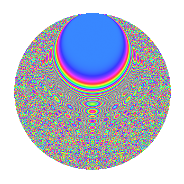# Properties

 Label 1849.2.eLevel 1849 Weight 2 Character orbit e Rep. character $$\chi_{1849}(78,\cdot)$$ Character field $$\Q(\zeta_{7})$$ Dimension 786 Sturm bound 315

# Related objects

## Defining parameters

 Level: $$N$$ $$=$$ $$1849 = 43^{2}$$ Weight: $$k$$ $$=$$ $$2$$ Character orbit: $$[\chi]$$ $$=$$ 1849.e (of order $$7$$ and degree $$6$$) Character conductor: $$\operatorname{cond}(\chi)$$ $$=$$ $$43$$ Character field: $$\Q(\zeta_{7})$$ Sturm bound: $$315$$

## Dimensions

The following table gives the dimensions of various subspaces of $$M_{2}(1849, [\chi])$$.

Total New Old
Modular forms 1074 1026 48
Cusp forms 810 786 24
Eisenstein series 264 240 24

## Trace form

 $$786q + 2q^{2} + q^{3} - 110q^{4} + q^{5} + 2q^{6} + 16q^{7} + 6q^{8} - 84q^{9} + O(q^{10})$$ $$786q + 2q^{2} + q^{3} - 110q^{4} + q^{5} + 2q^{6} + 16q^{7} + 6q^{8} - 84q^{9} - 7q^{10} - 5q^{11} - 11q^{12} + 3q^{13} + 15q^{14} + 9q^{15} - 78q^{16} - 5q^{17} - 13q^{18} + 2q^{19} - 15q^{20} - 8q^{21} - 6q^{22} - 3q^{23} + 4q^{24} - 46q^{25} - 3q^{26} - 29q^{27} - 21q^{28} - 15q^{29} + 16q^{30} + 23q^{31} + 36q^{32} - 20q^{33} - 12q^{34} - 4q^{35} + 184q^{36} + 18q^{37} + 27q^{38} - 5q^{39} - 19q^{40} - 26q^{41} + 38q^{42} - 166q^{44} + 26q^{45} - 16q^{46} - 22q^{47} - 11q^{48} + 46q^{49} - 12q^{50} + 24q^{51} - 7q^{52} + 11q^{53} - 56q^{54} - 19q^{55} - 23q^{56} + 9q^{57} + 25q^{58} - 38q^{59} - 47q^{60} - 31q^{61} - 45q^{62} - 9q^{63} - 14q^{64} - 19q^{65} - 37q^{66} + 21q^{67} - 10q^{68} - q^{69} + 26q^{70} - 54q^{71} + 29q^{72} - 12q^{73} - 12q^{74} - 11q^{75} - 32q^{76} + 21q^{77} - 24q^{78} + 38q^{79} + 38q^{80} + 117q^{81} + 3q^{82} + 18q^{83} + 28q^{85} - 158q^{87} + 28q^{88} - 22q^{89} + 76q^{90} + 4q^{91} + 36q^{92} + 28q^{93} + 23q^{94} - 3q^{95} - 44q^{96} - 14q^{97} - 26q^{98} - 9q^{99} + O(q^{100})$$

## Decomposition of $$S_{2}^{\mathrm{new}}(1849, [\chi])$$ into newform subspaces

The newforms in this space have not yet been added to the LMFDB.

## Decomposition of $$S_{2}^{\mathrm{old}}(1849, [\chi])$$ into lower level spaces

$$S_{2}^{\mathrm{old}}(1849, [\chi]) \cong$$ $$S_{2}^{\mathrm{new}}(43, [\chi])$$$$^{\oplus 2}$$

## Hecke characteristic polynomials

There are no characteristic polynomials of Hecke operators in the database